# Earthquake Parameter Prediction with Linear Regression

A Model for Earthquake Magnitude Prediction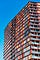Photo by Dan Gold on Unsplash

Python is one of the most common languages among those interested in data science. With its libraries , we can modify and use any type of data we want. With the help of Python and appropriate machine learning algorithms, we can create prediction models and graph the data as we want.

The steps we will take:

• Preparing the dataset
• Building a model with Linear Regression
• Visualisation with Matplotlib and Seaborn

# Let’s Prepare the Data

Let’s download the data in csv file format and start retrieving and editing the data with Pandas library. We can download earthquake data from open sources easily. For this, we can use database from AFAD or kaggle sites. I have a data including the earthquakes of Turkey between 1910–2017. I could read the data because files with `py`and `csv`extentions are on the same file path and I wrote that I would separate the data with a comma.

Let’s read the csv file with pandas:

`import pandas as pddata=pd.read_csv("earthquake.csv",sep=",")print(data)`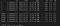Our data file is 2400x17 matrix

The result turned out like this. As you can see, we have a matrix file of 24007 rows and 17 columns in length. The columns are also distributed according to the title of the data. Let me explain them now:

• Id: order number of the earthquake
• Date: earthquake occurrence date
• Time: time of the earthquake
• Lat: latitude of the erthquake epicentre
• Long: longitude of the erthquake epicentre
• Country: country of the erthquake epicentre
• City: province of the occurred earthquake
• Area: region of the occurred earthquake
• Direction: direction of the earthquake signal
• Dist: district of the occurred earthquake
• Depth: depth of the ocurred earthquake (distance from the surface)
• Xm: the largest of the given magnitude values
• Md: magnitude depending on time
• Richter: Richter magnitude or the local magnitude (ML)
• Mw: moment magnitude
• Ms: surface wave magnitude
• Mb: body wave magnitude

This is how we look at the types of our data (as shown below). For Linear Regression, we will use only numerical data (float).

`data=data.info()print(data)`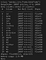Datas may be float or string.

There are null fields such as city, region, direction. I will read the processes I can use for this and leave the others. Let’s just write down the columns we want to read and look at the first 10 data.

`import pandas as pddata=pd.read_csv("earthquake.csv",sep=",")data=data[['id','lat','long','dist','depth','xm','md','richter', 'ms','mb']]data=data.head(10)print(data)`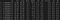Data including only numerical values

# Building a Prediction Model with Linear Regression

In machine learning, datas for analysis is divided into X (input) and y (output). I want to estimate the maximum magnitude value of xm parameter from the csv file I have. First, we translate the target variable that we will guess with the numpy library. We create the input data (X). We should delete the target variable that we will guess with the `drop` method. Let’s write axis = 1 since we are going to delete columns.

`import numpy as npy=np.array(veri['xm'])X=np.array(data.drop('xm',axis=1))`

We prepared the data for usage of the algorithm. We need to divide the existing data set into training and test data. At this step, we will use the scikit learn library. The ratio of test data is 20%. Let’s import the Linear Regression from the scikit learn library. Let’s take an example from this class and set up the model with the fit method using the training data . Due to the NaN values we can limit the data to avoid getting errors when using the fit method.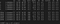NaN (not a number) data is not read and gives an error.
`#read latitude, longitude, depth and magnitude.data=data[['lat','long','depth','xm','md']]`

Yes, now let’s continue with the data with usable numerical values. We can look at the performance of the model with test data and training data. The accuracy rates of training data and test data should be close to each other. Here, because the score of the training data is larger than the test data, there is an overfitting situation.

`data=linear.score(X_test,y_test)print(data)df=linear.score(X_train,y_train)print(df)`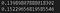Scores of taining and test values (very close)

Let’s look at the weights of the model’s coefficients (lat, long, depth, md) and constants (xm) that is, the predicted variable.

`#model weightsprint(linear.coef_)print(linear.intercept_)`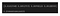Coefficients and prediction variable

Now we can make a prediction! Let’s give values for latitude, longitude, earthquake depth and magnitude due to the duration, and ask the estimation of the maximum magnitude, respectively.

`ddf=np.array([[40.05, 35.80, 10.0, 3.2]])ddf=linear.predict(ddf)print(ddf)`

Here are the results: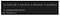Estimated magnitude is 4.12

Well, what does it mean? This the answer of what would be the the largest magnitude of an earthquake with latitude and longitude that are 40.05–35.80 (Turkey-Kocaeli ),10 km in depth, duration magnitude (md) as 3.2. We received an answer as 4.12. What if the model we have estimated this maximum size (xm) values we deleted earlier? Let’s make a selection from the data first.

`import pandas as pddata=pd.read_csv("earthquake.csv",sep=",")data=data[['date','time','lat','long','depth','city','xm',]]data=data.head(10)print(data)`

Let’s see the existing earthquake datas more clearly.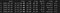Earthquake with 4.0 Magnitude in Kocaeli city

If we look at the data to examine it , the magnitude of the earthquake that took place in Kocaeli in 2007 (index 1) is shown as 4.0, with latitude 40.79, longitude 30.09, depth 5.2 km, and md = 3.8. Now, we can make predictions by using our model. For that we need to write the variables of the actual earthquake.

`data2=np.array([[40.79, 30.09, 5.2, 3.8]])df=linear.predict(data2)print(df`

We wrote the code, and the only data that has not been written is xm, which is the maximum magnitude we want to predict.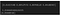Predicted magnitude

The earthquake that actually happened was 4.0 in the catalog and our estimate was found at the bottom as 4.099.

# Visualisation the Data

For this, we download the `matplotlib` and `seaborn` libraries.

`import matplotlib.pyplot as plt import seaborn as snf,ax = plt.subplots(figsize=(10, 10)) sn.heatmap(veri.corr(), annot=True, linewidths=.9, fmt= '.2f',ax=ax) plt.show()data.depth.plot(kind="line",grid=True,label="depth",linestyle=":",color="r")data.lat.plot(kind="line",grid=True,label="richter",linestyle="-",color="g")data.xm.plot(kind="line",grid=True,label="magnitude",linestyle="--",color="b")plt.legend(loc="best")  plt.title("depth-richter-magnitude")plt.show()`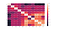Correlation of all the variables

We can draw a graph with parameters such as depth, Richter (ML) and xm (maximum magnitude) values. So, we can set the axis colors and titles from the code above.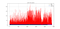Distribution of Depth-Richter-Magnitude (xm)

## Analytics Vidhya

Analytics Vidhya is a community of Analytics and Data…

## Sign up for Analytics Vidhya News Bytes

### By Analytics Vidhya

Latest news from Analytics Vidhya on our Hackathons and some of our best articles! Take a look.

By signing up, you will create a Medium account if you don’t already have one. Review our Privacy Policy for more information about our privacy practices.

Check your inbox
Medium sent you an email at to complete your subscription.

Written by

## Fatma Elik

Spatial Data Science | Machine Learning My projects are at https://github.com/FatmaElik | Linkedin: https://tr.linkedin.com/in/fatma-elik-399141b3

## Analytics Vidhya

Analytics Vidhya is a community of Analytics and Data Science professionals. We are building the next-gen data science ecosystem https://www.analyticsvidhya.com

Written by

## Fatma Elik

Spatial Data Science | Machine Learning My projects are at https://github.com/FatmaElik | Linkedin: https://tr.linkedin.com/in/fatma-elik-399141b3## Analytics Vidhya

Analytics Vidhya is a community of Analytics and Data Science professionals. We are building the next-gen data science ecosystem https://www.analyticsvidhya.com

Medium is an open platform where 170 million readers come to find insightful and dynamic thinking. Here, expert and undiscovered voices alike dive into the heart of any topic and bring new ideas to the surface. Learn more

Follow the writers, publications, and topics that matter to you, and you’ll see them on your homepage and in your inbox. Explore

If you have a story to tell, knowledge to share, or a perspective to offer — welcome home. It’s easy and free to post your thinking on any topic. Write on Medium